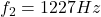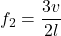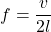## An open pipe, 0.29 m long, vibrates in the second overtone with a frequency of 1,227 Hz. In this situation, the fundamental frequency of the

Question

An open pipe, 0.29 m long, vibrates in the second overtone with a frequency of 1,227 Hz. In this situation, the fundamental frequency of the pipe, in SI units, is closest to:

in progress 0
2 months 2021-07-22T12:30:46+00:00 1 Answers 3 views 0

f = 409 Hz

Explanation:

We have,

Length of the open organ pipe, l = 0.29 m

Frequency of vibration of second overtone,It is required to find the fundamental frequency of the pipe. For the open organ pipe, the frequency of second overtone is given by :v is speed of sound

Let f is the fundamental frequency. It is given by :The relation between f and f₂ can be written as :So, the fundamental frequency of the pipe is 409 Hz.